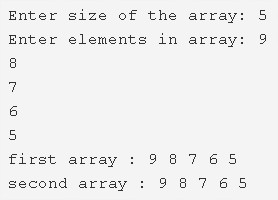# C Program To Copy All Elements From An Array | C Programs

C program to copy all elements from an array to another array – In this article, we will detail in on all the ways to copy all elements from an array to another array in C programming.

Suitable examples and sample programs have also been added so that you can understand the whole thing very clearly. The compiler has also been added with which you can execute it yourself. If you have any doubts related to C Program To Copy All Elements From An Array leave a comment here.

The ways used in this piece are as follows:

• Using Standard Method
• Using Function
• Using Recursion

As well know, arrays are a sequential arrangement of elements in a horizontal fashion. Arrays carry the basic form of information.In the image uploaded above, as it is mentioned, you have to enter the size of the array.

Afterwards, enter the elements in the given array.

The elements entered here as follows:

9 8 7 6 5

Thus, the elements will be copied exactly into the second array as:

9 8 7 6 5

Thus, the different ways to do so in C programming are as follows:

## Using Standard Method

1. Read the array size and store it into the variable n.

2) Read the entered elements using scanf() function and store the elements into the array a[]  using for loop fir(i=0;i<n;i++).Then all entered elements will be stored into the array a[].

3) To copy elements from the array a[] to the array b[]

for loop iterates through the string a[] with the structure for(i=0;i<n;i++).

b[i]=a[i]//copy the element of the array a[] to the array b[].

4) Print the elements of the first array, the second array using the print() function.

Then we can find that the second array also has the same elements like the first array.

Output:

### Using Function

1. The function copy() is the user defined function which copies the elements of one array into another array.

2) The main() function calls the copy() by passing a,b arrays and n as arguments to the function copy().

the function copy() will copy the array elements of a[] into the array b[] as b[i]=a[i] using for loop for(i=0;i<n;i++). After all iterations of for loop the elements of the array a[] will be copied into the array b[].

3) Print the both arrays using print() function.

Output:

#### Using Recursion

1. In this program, the function copy() is the recursive function which calls itself by increasing i value until the condition becomes false.

2) The main() function calls the function copy() by passing a,b arrays and size of the array n,0 as arguments.

3) The function copy() checks the condition i<size of the array,

If the condition is true then it copies a[i] into b[i] and calls itself as copy(a,b,n,++i) until the condition i<n becomes false.Every time it calls itself by increasing i value by 1.

4) After completion of the recursion, the main() will call the print() to print both array elements.

Output:

x

## C Program To Print Number Of Days In A Month | Java Tutoring

C program to input the month number and print the number of days in that ...